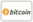Bookcover of Multi-Point Boundary Value Problems
Booktitle:

# Multi-Point Boundary Value Problems

## Multi Point Boundary Value Problems for Ordinary Differential Equations and Engineering Applications

LAP LAMBERT Academic Publishing (2012-12-04 )eligible for voucher
ISBN-13:

### 978-3-8383-6844-3

ISBN-10:
3838368444
EAN:
9783838368443
Book language:
English
Blurb/Shorttext:
This book proposes a semi-analytic technique to solve high-order non-linear multipoint boundary value problem for ordinary differential equation with non-local boundary conditions. The algorithm based on the two-point osculatory interpolation, essentially this is a generalization of interpolation using Taylor polynomials. The idea is to approximate a function y by a polynomial P in which values of y and any number of its derivatives at given points are fitted by the corresponding function values and derivatives of P. Illustrative examples are provided to demonstrate the efficiency, accuracy and simplicity of the proposed method in solving this type of boundary value problems, where the suggested solution is compared with the exact solution and the solution of iterative method, shooting method, uniform Haar wavelets and homotopy perturbation method (HPM). Also, we introduce some general observations about control of a residual and we proposed a new formula developed to estimate the error help reduce the accounts process and show the results are improved. The other aim of this book is to develop the existing MATLAB BVP ODE solver bvp4c and bvp6c into accurate solver.
Publishing house:
Website:
https://www.lap-publishing.com/
By (author) :
Mariam M. Hilal, Luma N. M. Tawfiq
Number of pages:
88
Published on:
2012-12-04
Stock:
Available
Category:
Mathematics
Price:
49.00 €
Keywords:
boundary value problems, Osculator Interpolation### Categories